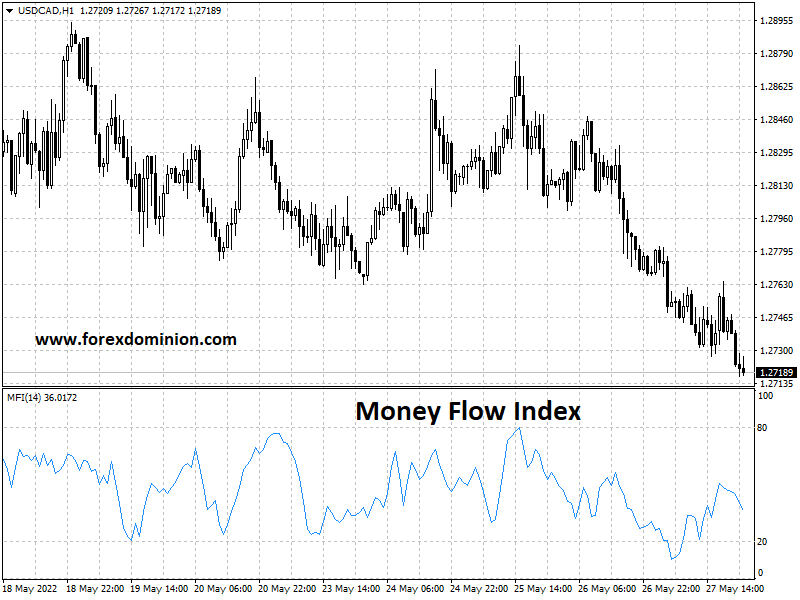# Money Flow Index Indicator | Overbought and oversold zones

The Money Flow Index Indicator, or MFI indicator, is a technical indicator used to identify overbought and oversold zones.

To understand the importance of this indicator, it is necessary to ask the following question: what moves the markets? The shortest answer maybe supply and demand.

If the demand for an instrument is high and there is little supply, the price will inevitably arise. If the opposite happens, the price will fall. Simple truth? But how can we assess what is happening with supply and demand? Especially in the Forex market, which is decentralized and consequently not very transparent.

One method that could work is to use price data to understand how money flows in and out of an instrument.

One of the most popular tools for doing this is the Money Flow Index indicator.Example of Money Flow Index of 14 periods (MFI 14)

## What is the Money Flow Index Indicator (MFI)

The MFI indicator looks at price changes and tick volume to determine if the flow is positive or negative. It is similar in some respects to the Accumulation/Distribution Indicator.

The Money Flow Index ranges from 0 to 100 levels. A basic use of the Money Flow Index is to indicate when the market is overbought or oversold.

• Oversold market: values below 20
• Overbought market: levels above 80

These levels are marked on the price chart as a gray dotted line. As a general rule, there is a higher probability of a price reversal at such points.

Now let’s take a look at how the values for the index are calculated.

## Calculation of the Money Flow Index indicator

There are several steps involved in calculating the MFI technical indicator. The good news is that you will not have to do them manually, since the indicator is configured automatically in many trading platforms like Metatrader 4. However, knowing what is behind this indicator will help you understand how it works.

The first step is to define a concept called typical price (TP), whose value is the arithmetic mean of the maximum, minimum, and closing price for the period in question.

Typical price = (H + L + C) / 3

Using this concept, we can go on and define the related concept of money flow (MF). We define this value by multiplying the typical price by the volume.

Money Flow: Typical Price x Volume

The next step is to compute the positive and negative money flows for N periods. A positive money flow will be one in which the TP is higher than that of the previous period. Consequently, negative money flow is any period when the TP is less than the previous period.

To calculate the positive money flow during N periods, we add the positive money flows during that period of time. Separately, we do the same thing for the negative flows, to calculate the negative flow of money over N periods. Dividing these two numbers gives us the money ratio (MR).

Money Ratio = (positive money flow)/(negative money flow)

Finally, we convert this to an index using the following equation:

MFI = 100 – 100/(1 + MR)

As we have already mentioned, if the above calculations seem complicated, don’t worry, the good news is that many trading platforms like Metatrader 4 have this indicator and will do the calculations for you.

But in order to use it, the first step is to have the MetaTrader 4 trading platform downloaded, it’s free!

## Using the Money Flow Index indicator in Metatrader 4

The MFI volume indicator in Metatrader 4 appears in the Indicators list and inside the ‘Volumes‘ folder, as you can see in the image below:

When you double-click on the indicator, the dialog box (see screenshot above) will appear, allowing you to configure the parameters according to your own preferences. Beyond the visual aspects of line color and thickness, the main settings are ‘Period’, ‘Fixed Minimum’ and ‘Fixed Maximum‘.

• Period is the value of N in the calculations shown above that defines the period of time over which we calculate positive and negative money flows. The default value is 14.
• The fixed minimum and fixed maximum define the values between which the MFI indicator will oscillate. 0 and 100 are the respective default values, and it is recommended that you trade with these values.

## How to interpret the Money Flow Index indicator

The image below shows an hourly GBP/USD chart to which we have added the Money Flow Index indicator:

The MFI indicator appears below the main chart. You can see the value of the Money Flow Index rising above the 80 levels in the green marked section. This indicates a period where the price is overbought, so you can see the price of GBP/USD starting to drop very soon.

## Conclusion

As we have seen, the Money Flow Index (MFI) indicator is a useful tool for measuring the buying and selling pressure caused by the flow of money, in and out of a particular market.

Using this relatively simple indicator it is possible to identify possible reversals at times when oversold or overbought values are displayed. This can be made even more effective by checking for divergent behavior between the price and the indicator.

The best way to find out which particular method is most effective for you is to try out the various techniques in the risk-free environment available with a demo trading account. That way, you can see what works best with real prices, in real-time, but without the fear of losing money while you’re still practicing. This will serve you well when you fully embark on your trading career.